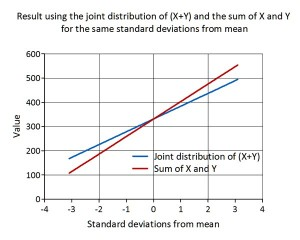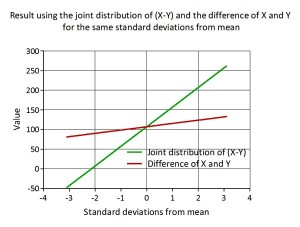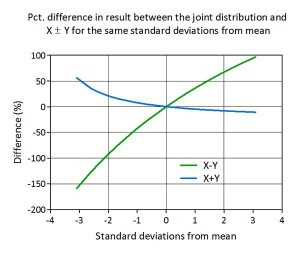# The fallacies of Scenario analysis

This entry is part 1 of 4 in the series The fallacies of scenario analysis

Scenario analysis is often used in company valuation – with high, low and most likely scenarios to estimate the value range and expected value. A common definition seems to be:

Scenario analysis is a process of analyzing possible future events or series of actions by considering alternative possible outcomes (scenarios). The analysis is designed to allow improved decision-making by allowing consideration of outcomes and their implications.

Actually this definition covers at least two different types of analysis:

1. Alternative scenario analysis; in politics or geo-politics, scenario analysis involves modeling the possible alternative paths of a social or political environment and possibly diplomatic and war risks – “rehearsing the future”,
2. Scenario analysis; a number of versions of the underlying mathematical problem are created to model the uncertain factors in the analysis.

The first addresses “wicked” problems; ill-defined, ambiguous and associated with strong moral, political and professional issues. Since they are strongly stakeholder dependent, there is often little consensus about what the problem is, let alone how to resolve it. (Rittel & Webber,1974)

The second cover “tame” problems; that has well-defined and stable problem statements and belongs to a class of similar problems which are all solved in the same similar way. (Conklin, 2001) Tame however does not mean simple – a tame problem can be very technically complex.

Scenario analysis in the last sense is a compromise between computational complex stochastic models (the S&R approach) and the overly simplistic and often unrealistic deterministic models. Each scenario is a limited representation of the uncertain elements and one sub-problem is generated for each scenario.

Best Case/ Worse Case Scenarios analysis.
With risky assets, the actual cash flows can be very different from expectations. At the minimum, we can estimate the cash flows if everything works to perfection – a best case scenario – and if nothing does – a worst case scenario.

In practice, each input into asset value is set to its best (or worst) possible outcome and the cash flows estimated with those values.

Thus, when valuing a firm, the revenue growth rate and operating margin etc. is set at the highest possible level while interest rates etc. is set at its lowest level, and then the best-case scenario value is computed.

The question now is – if this really is the best (or worst) value or if let’s say a 95% (5%) percentile is chosen for each input – will that give the 95% (5%) percentile for the firm’s value?

Let’ say that we in the first case – (X + Y) – want to calculate entity value by adding ‘NPV of market value of FCF’ (X) and ‘NPV of continuing value’ (Y). Both are stochastic variables, X is positive while Y can be positive or negative.  In the second case – (X – Y) – we want to calculate the value of equity by subtracting value of debt (Y) from entity value (X). Both X and Y are stochastic, positive variables.

From statistics we know that for the joint distribution of (X ±Y) the expected value E(X ±Y) is E(X) ± E(Y) and that Var(X ± Y) is Var(X) + Var(Y) ± 2Cov(X,Y). Already from the expression for the joint variance we can see that this not necessarily will be true. However the expected value will be the same.

We can demonstrate this by calculating a number of percentiles for two normal independent distributions (with Cov(X,Y)=0, to make it simple) and add (subtract) them and plot the result (red line) with the same percentiles from the joint distribution  – blue line for (X+Y) and green line for (X-Y).As we can see the lines for X+Y only coincides at the expected value and the deviation increases as we move out on the tails. For X-Y the deviation is even more pronounced:Plotting the deviation from the joint distribution as percentage from X Y, demonstrates very large relative deviations as we move out on the tails and that the sign of the numerical operator totally changes the direction of the deviations:Add to this, a valuation analysis with a large number of:

1. both correlated and auto-correlated stochastic variables,
2. complex calculations,
3. simultaneous equations,

and there is no way of finding out where you are on the probability distribution – unless you do a complete Monte Carlo simulation. It is like being out in the woods at night without a map and compass – you know you are in the woods but not where.

Some advocates scenario analysis to measure risk on an asset using the difference between the best-case and worst-case. Based on the above this can only be a very bad idea, since risk in the sense of loss is connected to the left tail where the deviation from the joint distribution can be expected to be the largest. This brings us to the next post in the series.

### References

Rittel, H., and Webber, M. (1973). Dilemmas in a General Theory of Planning. Policy Sciences, Vol. 4, pp 155-169. Elsevier Scientific Publishing Company, Inc: Amsterdam.

Conklin, Jeff (2001). Wicked Problems. Retrieved April 28, 2009, from CofNexus Institute Web site: http://www.cognexus.org/wpf/wickedproblems.pdfS@R develops models for support of decision making under uncertainty. Taking advantage of recognized financial and economic theory, we customize simulation models to fit specific industries, situations and needs.

### 3 Enlightened Replies

1.bearawap says:

Hi, discriminative posts there 🙂 through’s for the compelling advice

2.IsotsHoth says:

Hi, Congratulations to the site owner for this marvelous work you’ve done. It has lots of useful and interesting data.

3.Gekko says:

Very nice explanation – thanks !

Top